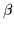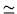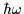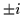Next: Coulomb effects and isospin Up: Single-particle structures at N=Z=16 Previous: Single-particle structures at N=Z=16

## Deformed shell gaps

The ground state of the 32S nucleus, obtained within the HF approach with the SLy4 force, corresponds to a spherical-shape configuration that contains, on top of the closed 16O core, the 1d5/2 and 2s1/2 orbitals filled, both for the neutrons and protons. With an increase in the prolate deformation, the negative-parity Nilsson orbitals originating from the spherical 16O core stay occupied (see Fig. 2.21a in Ref.  for a qualitative illustration). The same is true for the positive-parity valence orbitals, except for the orbital 5/2 (the up-sloping extruder orbital), which originates from the spherical 1d5/2 shell, and rapidly grows up in energy with increasing deformation. After this orbital is crossed by the 1/2 orbital (the down-sloping intruder orbital), which originates from the spherical 1f7/2 shell, one obtains a large, about 2.5MeV gap that corresponds to a SD configuration in the 32S nucleus. Therefore, the SD states in such a light system as 32S, formally correspond to the 4p-4h excitation with respect to the spherical ground state. However, our calculations presented in detail below indicate that the SD configurations have extremely large quadrupole deformations,0.7, and the implied structures of the SD, ND (normal deformed), or spherical wave functions have so little similarities that the notion of particle-hole excitations with respect to the spherical ground-state is not very useful.

Figures 1 and 2 show the neutron and proton single-particle routhians, as functions of the cranking frequency. One can see that over a very large range of the rotational frequencies, there exists an important gap in the single-particle HF spectrum at the neutron and proton numbers N=Z=16. By definition, in the underlying 32S SD configuration all the neutron and proton levels lying below the gaps at N=16 and Z=16 are occupied, and all those above the gaps are empty.

As a result of the presence of those large gaps in the single-particle 32S proton and neutron spectra, we refer to the corresponding lowest-energy SD state as to the magic SD configuration.

A characteristic result visible from Figs. 1 and 2 is that the over-all single-particle structure of the HF orbitals near the Fermi level is remarkably simple. First of all, the dependence of the single-particle routhians on the rotational frequency is very regular, and there is only one clear-cut crossing caused by the down-sloping routhians 1/2(r=-i), originating from the N0=4 shell. Second, the density of levels appearing in the figures is very low as compared, e.g., to those in the mass A150 region of SD nuclei. The negative parity states are represented only by two N0=3 intruder orbitals 1/2(r=) below, and two intruder orbitals 3/2(r=) above the Fermi level. Similarly, in the positive parity there are only two states 1/2(r=) below, and two extruder states 5/2(r=) above the Fermi level. Signature splitting of the extruder states 5/2(r=) is very weak, because they carry high K=5/2 angular momentum projection, whereas splitting between the intruder levels 3/2(r=) is more pronounced. It becomes well visible at rotational frequencies of about 0.8MeV. Below the Fermi level, orbitals 1/2(r=) and 1/2(r=) have K=1/2, hence both are strongly split.Next: Coulomb effects and isospin Up: Single-particle structures at N=Z=16 Previous: Single-particle structures at N=Z=16
Jacek Dobaczewski
1999-07-27